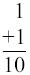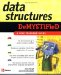# Data and Memory

Data used by your program is stored in memory and manipulated by various data structure techniques, depending on the nature of your program. Let s take a close look at main memory and how data is stored in memory before exploring how to manipulate data using data structures.

Memory is a bunch of electronic switches called transistors that can be placed in one of two states: on or off. The state of a switch is meaningless unless you assign a value to each state, which you do using the binary numbering system.

The binary numbering system consists of two digits called binary digits (bits): zero and one. A switch in the off state represents zero, and a switch in the on state represents one. This means that one transistor can represent one of two digits.

However, two digits don t provide you with sufficient data to do anything but store the number zero or one in memory. You can store more data in memory by logically grouping together switches. For example, two switches enable you to store two binary digits, which gives you four combinations, as shown Table 1-1, and these combinations can store numbers 0 through 3. Digits are zero-based , meaning that the first digit in the binary numbering system is zero, not 1. Memory is organized into groups of eight bits called a byte , enabling 256 combinations of zeros and ones that can store numbers from 0 through 255.

Table 1-1: Combinations of Two Bits and Their Decimal Value Equivalents

Switch 1

Switch 2

Decimal Value

1

1

1

2

1

1

3

### The Binary Numbering System

A numbering system is a way to count things and perform arithmetic. For example, humans use the decimal numbering system, and computers use the binary numbering system. Both these numbering systems do exactly the same thing: they enable us to count things and perform arithmetic. You can add, subtract, multiply, and divide using the binary numbering system and you ll arrive at the same answer as if you used the decimal numbering system.

However, there is a noticeable difference between the decimal and binary numbering systems: the decimal numbering system consists of 10 digits (0 through 9) and the binary numbering system consists of 2 digits (0 and 1).

To jog your memory a bit, remember back in elementary school when the teacher showed you how to carry over a value from the right column to the left column when adding two numbers? If you had 9 in the right column and added 1, you changed the 9 to a 0 and placed a 1 to the left of the 0 to give you 10:The same carry over technique is used when adding numbers in the binary numbering system except you carry over when the value in the right column is 1 instead of 9. If you have 1 in the right column and add 1, you change the 1 to a 0 and place a 1 to the left of the 0 to give you 10:Now the confusion begins. Both the decimal number and the binary number seem to have the same value, which is ten. Don t believe everything you see. The decimal number does represent the number 10. However, the binary number 10 isn t the value 10 but the value 2.

The digits in the binary numbering system represent the state of a switch. A computer performs arithmetic by using the binary numbering system to change the state of sets of switches.Data Structures Demystified (Demystified)
ISBN: 0072253592
EAN: 2147483647
Year: 2006
Pages: 90

Similar book on Amazon